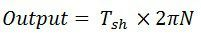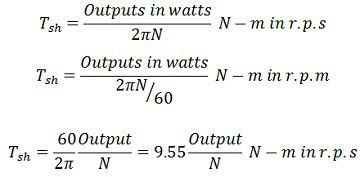# Shaft Torque

In a DC Motor whole of the electromagnetic torque (T) developed in the armature is not available on the shaft. A part of it is lost to overcome the iron and mechanical (friction and windage) losses. Therefore, shaft torque (Tsh) is somewhat less than the torque developed in the armature.

Definition: Thus, in the case of DC motors, the actual torque available at the shaft for doing useful mechanical work is known as Shaft Torque. It is so called because it is available on the shaft of the motor. It is represented by the symbol Tsh. The output of the motor is given by the equation shown below where Tsh is the shaft torque in r.p.s and the N is the rotation of the motor in r.p.m. The shaft torque is expressed asThe difference between the armature torque and the shaft torque ( Ta – Tsh ) is known as the lost torque and is due to the formation of the torque.

Brake Horse Power (B.H.P)

In the case of the motor, the mechanical power available at the shaft is known as Brake Horse Power. If Tsh is the shaft torque in Newton Meter and N is the speed in r.p.m then,The output brake horsepower is given by the equation (1) shown above.